# eryar

posts - 439, comments - 590, trackbacks - 0, articles - 0

eryar@163.com

Key Words. Function Set, Function Set Root, Newton Raphson Algorithm

### 1.Introduction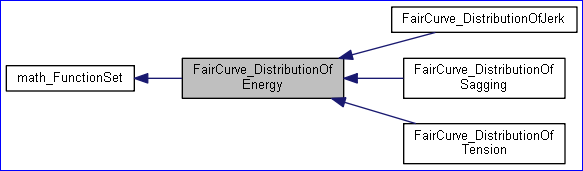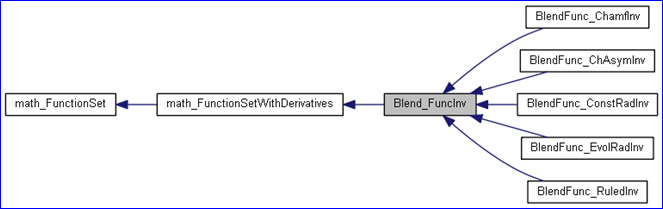### 2.Function Set Definition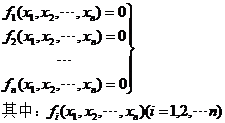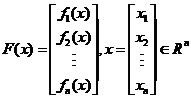l NbVariables()：变量的个数，即末知量的个数；

l NbEquations()：方程的个数，即方程组中有几个方程；

l Value(const math_Vector&X, math_Vector& F)：方程组的值，即代入变量每个方程的值；

### 3.Function Set Root Algorithm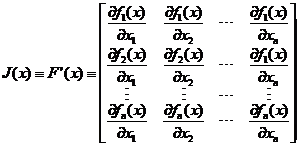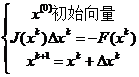l Derivatives(const math_Vector& X, math_Matrix& D)：一阶偏导数值，即计算Jacobi矩阵；

l Values(const math_Vector& X, math_Vector& F, math_Matrix& D)：计算方程的值及一阶偏导数矩阵Jacobi矩阵。

### 4.Code Example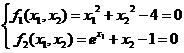#include <math_FunctionSet.hxx>
#include
<math_FunctionSetWithDerivatives.hxx>
#include
<math_FunctionSetRoot.hxx>
#pragma comment(lib,
"TKernel.lib")
#pragma comment(lib,
"TKMath.lib")
class MyFunctionSet : public math_FunctionSetWithDerivatives
{
public:

virtual Standard_Integer NbVariables() const
{

return 2;
}

virtual Standard_Integer NbEquations() const
{

return 2;
}

virtual Standard_Boolean Value(const math_Vector& X, math_Vector& F)
{
F(
1= X(1* X(1+ X(2* X(2- 4.0;
F(
2= Pow(M_E, X(1)) + X(2- 1.0;

return Standard_True;
}

virtual Standard_Boolean Derivatives(const math_Vector& X, math_Matrix& D)
{

// matrix D is Jacobi matrix.
D(11= 2.0 * X(1);
D(
12= 2.0 * X(2);
D(
21= Pow(M_E, X(1));
D(
22= 1.0;

return Standard_True;
}

virtual Standard_Boolean Values(const math_Vector& X, math_Vector& F, math_Matrix& D)
{
Value(X, F);
Derivatives(X, D);

return Standard_True;
}
private:
};
void test()
{
MyFunctionSet aFunctionSet;
math_FunctionSetRoot aSolver(aFunctionSet);
math_Vector aStartingPoint(
12);

// 1. (1.0, 1.0)
aStartingPoint(1= 1.0;
aStartingPoint(
2= 1.0;
aSolver.Perform(aFunctionSet, aStartingPoint);

if (aSolver.IsDone())
{
aSolver.Dump(std::cout);
}

// 2. (1.0, -1.0)
aStartingPoint(1= 1.0;
aStartingPoint(
2= -1.0;
aSolver.Perform(aFunctionSet, aStartingPoint);

if (aSolver.IsDone())
{
aSolver.Dump(std::cout);
}
}
int main(int argc, char* argv[])
{
test();

return 0;
}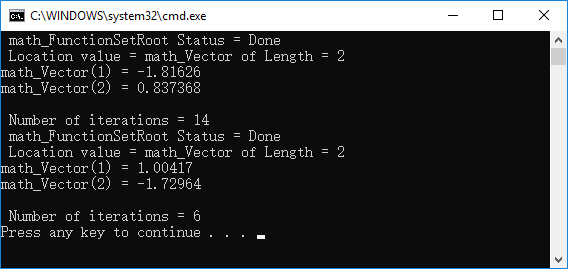### 5.Conclusion